### Diary for 01:090:101:21, Experimental Math, fall 2008

#### The nineth meeting, 11/3/2008

Most students who take a math course in college will take some sort of calculus course. Just look at the Rutgers course schedules -- the Math Department's dominating teaching activity is calculus. Calculus is about the theory and applications of derivative and integral. I'd like to draw some pictures and help you understand one of these words. And experimentation will reveal that things are not as they seem, both theoretically and practically.

A random (polynomial) example

Consider the function which is defined by this equation:
f(x)=x7-17x4+5x2+3x-12
Let's look at some simple graphs of y=f(x).
 plot(f(x),x=-2..3,thickness=2,color=black); The graph on [-2,3] I made the thickness larger (the default is 1) so I could see it better and I made the color of the curve black because that seemed saner (?) than red, which is what's used if no color is specified.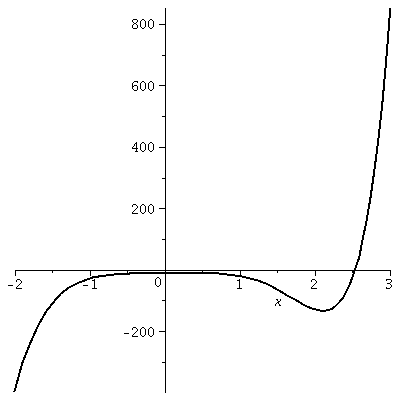We will now "zoom in" on this curve. The Maple software when given an interval automatically (unless you advise it otherwise!) tries to recenter the graph and to use the window supplied as well as possible. So if a horizontal interval is given, the appropriate vertical interval is taken to show the function's graph as well as possible. plot(f(x),x=1..2,thickness=2,color=black); The graph on [1,2] Probably you can see where this graph came from on the picture above. It retains a slight amount of curviness.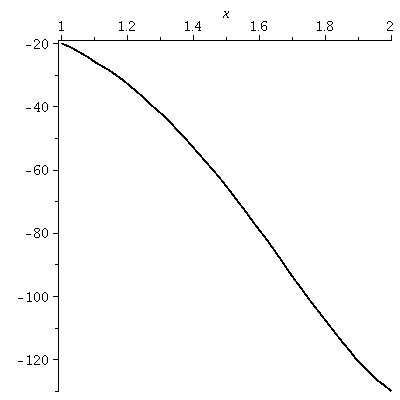plot(f(x),x=1.6..1.7,thickness=2,color=black); The graph on [1.6,1.7] Now something interesting is happening because of the simplicity shown. We have zoomed in again. To me there is still a slight bend in the curve but the picture is closer to a straight line.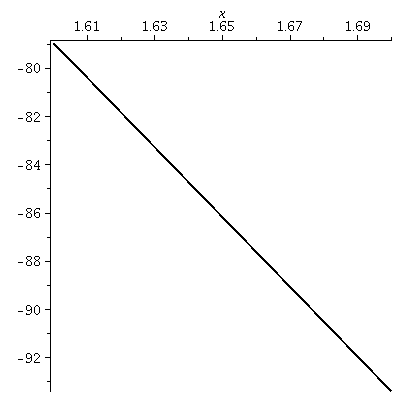plot(f(x),x=1.65..1.66,thickness=2,color=black); The graph on [1.65,1.55] Again we go in. To me what's shown is visually indistinguishable from a straight line. Further zooming, which you can try, doesn't lead to pictures which are different from what's shown here.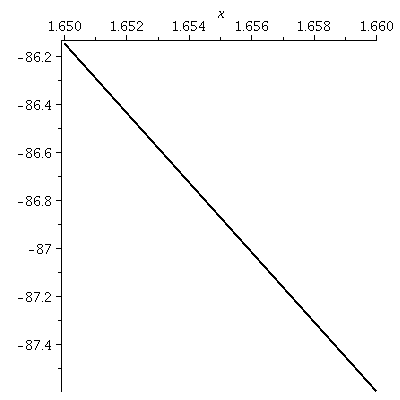Local linearity
With the help of the machine, we can see that under a suitable "microscope" the graph becomes a straight line. Sometimes people say that the graph is locally linear. The word "locally" means a heck of a lot of magnification, of course. The slope of the line shown is called the derivative. If you give me almost any function defined by a formula using familiar "things" then, with sufficient magnification, the graph will change into a line.

Lots of magnifications for nice functions yield straight lines. That is the idea behind a whole bunch of calculus. It is remarkable that these properties of functions were deduced historically with much less evidence then what can be generated with Maple in a short time. This is remarkable. But let's try another example.

 Absolute value I want to graph a function that is familiar but probably not admired by many, especially those who meet it and are annoyed by it in algebra. The function is absolute value, and the absolute value of x is usually written |x|. The official definition of |x| is clumsy. Here is it is:```      x if x≥0      |x| =       -x if x<0```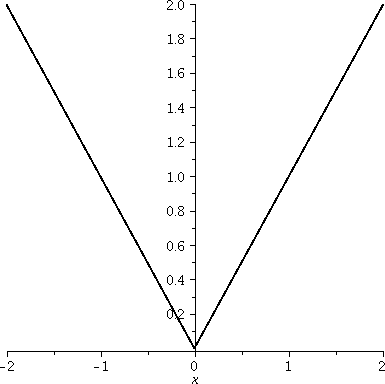plot(|x|,x=-2..2,thickness=2,color=black); The graph on [-2,2] Some of the irritations of Maple's graphs are visible here. Maybe they are not irritations -- they are features. Let's see: first, the corner of |x| seems to be floating above the horizontal axis and doesn't go through (0,0). If you look critically at the graph, though, you will see that the corner is at (0,0). The horizontal line is slightly below y=0. But there's another, more subtle "error". The aspect ratio of the graph is wrong, and the tilt of the lines is wrong. The graph is 4 units wide and only 2 units high. (Aspect ratio is the way horizontal and vertical units are related.) The x and -x parts of the graph should have slope equal to 1 and -1, and the slopes shown don't seem to be these quantities (for example, the rays should be perpendicular to each other, which is not how they are displayed). This is the default view for graphs produced by Maple. It is called unconstrained scaling. We can fix this if we wish. plot(|x|,x=-2..2,thickness=2,color=black,scaling=constrained);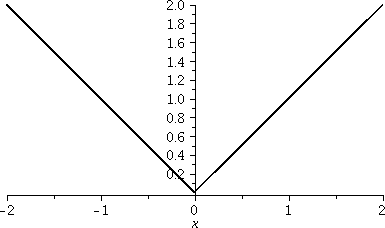The graph on [-2,2] again You can make the option scaling=constrained part of the command or it can be changed by clicking on the mouse when the cursor is over the image. The angles and aspect ratio are now displayed "exactly". plot(|x|,x=-.005..(.005),thickness=2,color=black,scaling=constrained);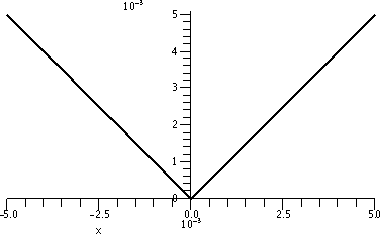The graph on [-.005,.005] Would I lie to you? This really, really, really is the Maple graph of |x| on a tiny interval centered around 0. It surely is not becoming a straight line. It surely does not seem to be different -- in fact, it seems to be self-similar, and the corner here matches up with the corner in the previous graph.

Not locally linear!
The absolute value function is not locally linear. There is no magnification which will straighten it out around (0,0). For those of you who haven't taken a calculus course (and you should check with those who have!) note that this awkwardness is handled in such courses by just throwing out the function. Don't think about it!

Let's make things a bit worse. Here are more pictures.

 Here is a graph of |x–4| on the interval [-5,5]. The "–4" moves the corner to the right. That – moves the picture right has always been slightly irritating to me. That's the way it is, though.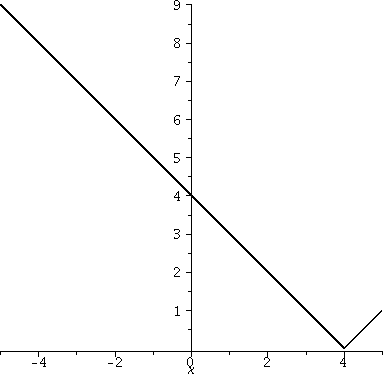Here is a graph of –|x+3| on [-5,5]. Now the corner has been moved to the left, and is at -3. The –, the minus sign in front, causes the corner to open in the negative y direction: the graph is flipped over the y-axis.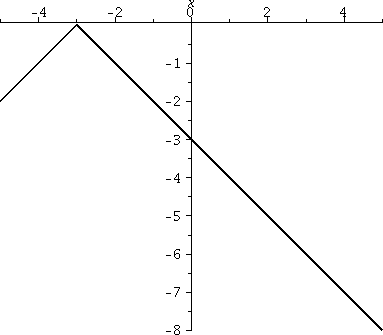Now let me consider a more complicated function, which is a sum of multiples of "moved" absolute values. After all, polynomials are a sum of multiples of powers of x. This function is

4|x-4|-5|x-2|+7|x|-8|x+2|.
 Constrained To the right is a picture of the graph in constrained mode: the "truth". Maybe this, while true, is not very informative. Here the domain is [-5,5] again, and the range of the graph is from less than -30 to almost 20 (I learn this by looking at the numbers on the vertical axis). So the aspect ratio of this true view is 5 to 1! I find this graph difficult to learn much from. It seems squeezed.Unconstrained Now the graph is displayed "unconstrained", and although maybe the angles are not accurate, at least we can see more clearly some of the other qualitative aspects of the graph. To the right is a picture of the graph in unconstrained mode. Here the graph has been widened, so there is a distortion. But now ... I hope you can see that there are 4 corners, and I bet that the function can't be "locally linearized" at those 4 points. You'd better not study this function in calculus -- it isn't nice.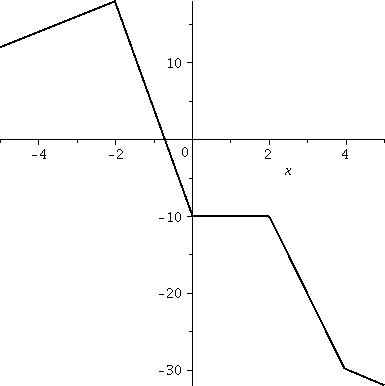Where do you see such graphs? Not in a calculus course -- they are horrible and strange and hard to think about. But you will see them in almost any daily newpaper and on lots of web pages, when financial markets report prices.

History
In 1827, the biologist Robert Brown microscopically observed the motion of pollen in water. The pollen seemed to jump bizarrely, in strange and jagged paths, very different from the smooth motion of, say, a cannon ball in a parabolic trajectory. Observation of dust particles gave the same sort of results, so the motion couldn't be attributed to some sort of life-force in the pollen grains. This movement of the particles was named Brownian motion. The doctoral thesis of Bachelier in 1900 connected Brownian motion with variations in stock and option markets. Such jagged graphs typically appear in many financial reports. One of Einstein's famous results of 1905 explained Brownian motion using probability -- the particles of dust move as a result of random molecular collisions, and the molecules move because of heat.

The paths typically are not smooth curves, and are usually not differentiable! There is no magnification which will make these curves appear locally like straight lines. The functions involved are not locally linear. There is a sort of self-similarity, though, because when the paths are magnified, more roughness appears and the smaller scale views seem as bad as the large-scale view. For more discussion, here is some history and intuitive metaphor. Here is a very clever moving applet (?) which may make the idea of Brownian motion clearer. You may need to look at it for a while to understand what's being shown.

In the last 20 or 30 years, Brownian motion and related topics have been extensively studied by mathematicians and physicists, and non-differentiable functions are standard tools in mathematical finance and other areas!

Much more interesting behavior can occur, and displaying some of this behavior to you is my goal today. I will try to build the display gradually. Please realize that the functions and graphs you have looked at in most of your courses are actually very special -- indeed, most functions are much more like what I'm about to describe than all of the so-called standard functions of calculus. We will go step-by-step.

round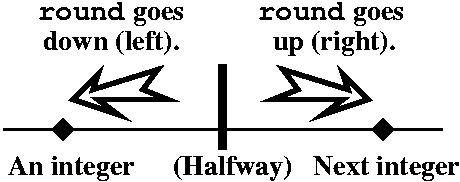Maple has a built-in function called round. Probably you can tell me what round does if I show you some values:
round(.9)=1   round(1.2)=1    round(.2)=0
And a few negative (?) values:
round(-.3)=0   round(-1.7)=-2
The picture to the right is supposed to help you understand what round does.

The sawtooth function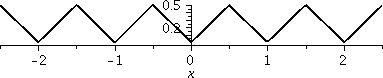round(x) computes the nearest integer to x. We can create the sawtooth function with round(x). To the left is a graph of |round(x)-x|. The graph shows you why this is called the sawtooth function. This function has many real applications in engineering, but I want to "appreciate" the picture and try to explain it. Since round(x) gets the closest integer, and a number can't be any farther away from the closest integer than 1/2, the value of this function is between 0 and 1/2. Also, the function is always positive because of the outer absolute value. It gives the distance to the nearest integer, and repeats itself in every integer interval, so from now on I'll only show graphs in the interval [0,1]. The other intervals repeat this picture. Some of these pictures will be difficult to understand, or you will want to deny they exist. That's o.k.: many 19th century mathematicians and physicists had the same feelings.> st:=x->|round(x)-x|;
 This defines the sawtooth function as st so that we can refer to it more easily.
To the right is a plot of st. The horizontal scale of the graph is [0,1] and the vertical scale, [0,.5].
These options were specified:
color=black,
thickness=2,
scaling=constrained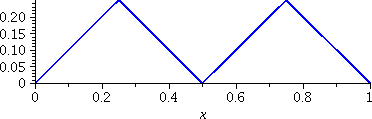This is a graph of (1/2)st(2x). The horizontal scale of the graph is [0,1] and the vertical scale, [0,.25].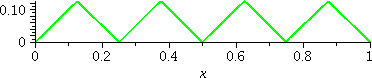This is a graph of (1/4)st(4x). The horizontal scale of the graph is [0,1] and the vertical scale, [0,.125]. 4 is 22.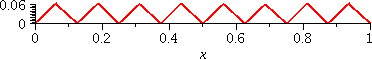This is a graph of (1/8)st(8x). The horizontal scale of the graph is [0,1] and the vertical scale, [0,.0625]. 8 is 23.

Now comes the real thing. Graphs of several functions are displayed together. We are adding up what was shown before.

 st(x) is in black. st(x)+(1/2)st(2x) is in green. st(x)+(1/2)st(2x)+(1/4)st(4x) is in blue. st(x)+(1/2)st(2x)+(1/4)st(4x)+(1/8)st(8x) is in red.Name and graph These sums are all approximations of what's called the Takagi function, named after a Japanese mathematician (more historical information is below). So let me jump more deeply and display a graph of add((1/2^j)*st((2^j)*x),j=0..10),x=0..1) and tell you that this sum is the first 10 terms (no, 11, sorry!) of the Takagi function.Here are some more pictures. I chose the interval [.362,.363] as a fairly random interval and I would like to investigate what happens "microscopically" there. I also defined
so that I could write the plotting instructions more easily.

 Looking at one approximation of the Takagi graph on a small interval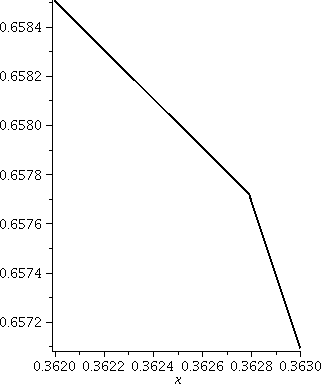To the right is a graph of T(x,10) on the interval [.362,.363]. On the big scale of the whole unit interval, with the picture shown above, the function looked curvy. Here the length of the interval is .001, and you can "see" a corner. The sum for T(x,10) adds up terms which steadily get smaller, and the smallest has bumps which are 2-10≈.0098, about the same size. So perhaps we should not be surprized we see a corner. But Mr. Takagi wants us to add up more terms, more and more and more terms! A closer approximation of the Takagi function on this tiny interval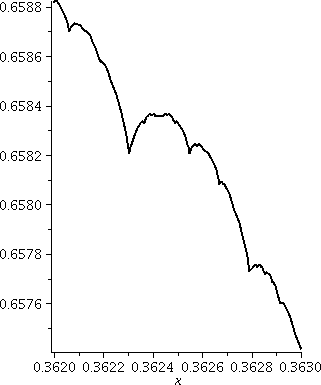Here is a graph of T(x,20) on the interval [.362,.363]. Now we've made the terms and the sawtooths smaller. The scale of the last term is 2-20≈.000001, and this is one-thousandth the width of the window we contemplate to the right. There are lots of corners in this window. You can continue to play with zooming and with adding up more terms. Now I will tell the entire story.

The Takagi function and its graph
Here is the official definition of the "whole" Takagi function.

T(x)=st(x)+(1/2)st(2x)+(1/4)st(4x)+(1/8)st(8x)+ and so on ...

From the point of view of modeling and understanding Brownian motion and other real phenomena, the phrase "and so on ..." is probably the most important part of the definition. We add up infinitely many successively smaller sawtooth functions. There are bumps being placed everywhere so that no matter how much you magnify the graph, there will be no local linearity showing at any point of the graph. This function, in the language of calculus, is not differentiable at every point: terrible, terrible, terrible. That it happens to be a better model of reality for many situations than polynomials, which are much beloved and computed, is perhaps more a statement about human culture.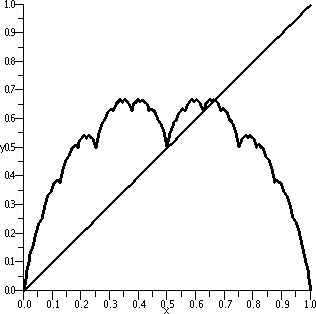The graph of the Takagi function is what's called a fractal. The definition of this word is imprecise (please look at the link given for extensive discussion and a large variety of examples). Most definitions of fractals include some kind of self-similarity. This means that the object looks the same at different scales or magnifications. The graph of the Takagi function has a sort of self-similarity. To the right is a graph of an approximation (of course since I don't have time to wait for a computer to add up an infinite number of terms!) of the Takagi function. There is also a straight line shown: y=x. In the first half of the interval, for x between 0 and .5, the difference between the line and the Takagi function is precisely similar to the graph of the Takagi function on [0,1].

Pictures of this self-similarity
Here is a display the self-similarity just mentioned. Below there are graphs of two different functions on the interval [0,.5]. On the left, in magenta, is T(x,10)-x. On the right, in brown, is a graph of (1/2)T(2x,10). They seem to look alike.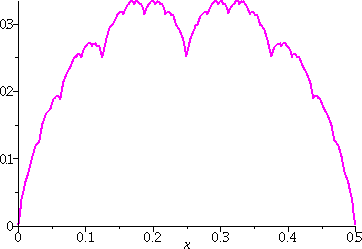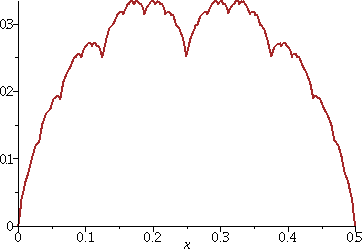History
After the initial observations of Brown, most mathematicians tried diligently to ignore the possibilities. The first person who may have attempted to imagine pictures like those we've drawn today was a mathematician named Bolzano whose work was mostly forgotten (!). In the late 19th century, the very famous European mathematicians Riemann and Weierstrass gave academic credibility to the "construction" of functions which are crinkly (not locally linear, not differentiable!) at every point. The specific function described here was described (discovered?) by the Japanese mathematician Teiji Takagi (1875--1960) in 1903. Several sentences from his biography should be quoted:

He attended primary school in Kazuya Village before going to middle school in Gifu entering this second stage of his education in 1886. At that time there were no mathematics texts written in Japanese so the pupils studying mathematics had to use English texts.
Try to imagine Takagi learning math at age 11 from books in English -- and include in your imagining that English and Japanese are known to be mutually difficult to understand.

Takagi's function has apparently been rediscovered frequently. It seems to be a natural and simple example. David Tall, an English mathematics educator, has written an exposition about the Takagi function (aimed at teachers). It is available here. Another article by him discussing the function is here. Of course, there is a Wikipedia article.You can contrast the Takagi function with one of the examples of Weierstrass, much better known, which is a sum of sine functions (instead of sawtooth functions). This example is add((1/2^j)*sin((3^j*x,j=1..N) (where we imagine that the N is ∞). The picture to the right is a graph of this function when N=30 on the interval [0,2π].

One further historical remark
Here is a quote from page 233, Scenes from the History of Real Functions by Fedor Andreevich Medvedev, Springer 1991, which I accessed through a Google link.

By the beginning of the twentieth century the representatives of the traditional view had begun to yield their positions, though not completely. It was difficult to combat obvious facts and a curious temporary exit was found. Functions having singularities ... came to be regarded as annoying exceptions among the "good" functions, as certainly pathological phenomena in the basically healthy body ... it was even generously decided to study these diseased tumors ...

It turned out in the 1930's that this picture did not correspond to the actual state of affairs. The class of continuous nowhere differentiable functions turned out to be immeasurably richer than the class of differentiable functions and it was rather functions of the latter type that were "pathological". A curious situation arose, when it turned out that the continuous functions that had been studied by mathematicians for centuries, those that were used to describe the phenomena of the external world, belong to a negligibly small class of continuous functions.

 More pictures You can play with functions and summing and plotting and get many strange pictures. Here: > g:=x->frac(x)*(1-frac(x));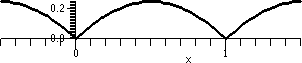This gets the top piece of a parabola on [0,1] and repeats it in every integer interval. A picture of the graph on [-.5,1.5] is shown.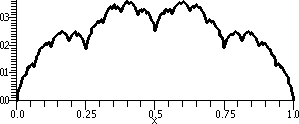> Q:=(x,n)->add((1/3^j)*g((4^j)*x),j=0..n); This adds up rescaled copies of g. The internal/external scalings in the sum don't need to be the same. A graph of Q(x,10) on [0,1] is shown.